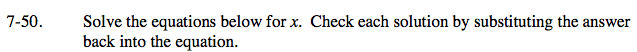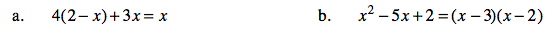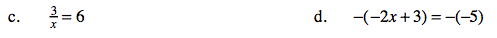### Home > AC > Chapter 7 > Lesson 7.1.5 > Problem7-50

7-50.
1. Solve the equations below for x. Check each solution by substituting the answer back into the equation. Homework Help ✎

1. 4(2 − x) + 3x = x

2. x2 − 5x + 2 = (x − 3)(x − 2)

3.= 6

4. −(−2x + 3) = −(−5)For more help solving equations, see problem 6-107.x = 4x = 0.5